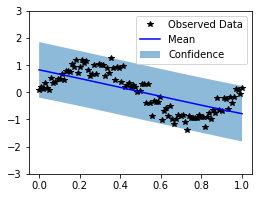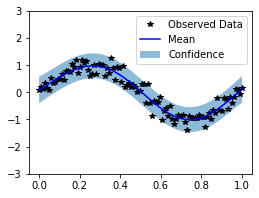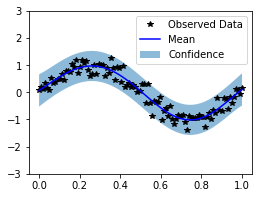# Implementing a custom kernel in GPyTorch¶

In this notebook we are looking at how to implement a custom kernel in GPyTorch. As an example, we consider the sinc kernel.

:

import math
import torch
import gpytorch
from matplotlib import pyplot as plt


Before we start, let’s set up some training data and convenience functions

:

import os
smoke_test = ('CI' in os.environ)
training_iter = 2 if smoke_test else 50

# Training data is 100 points in [0,1] inclusive regularly spaced
train_x = torch.linspace(0, 1, 100)
# True function is sin(2*pi*x) with Gaussian noise
train_y = torch.sin(train_x * (2 * math.pi)) + torch.randn(train_x.size()) * math.sqrt(0.04)

# Wrap training, prediction and plotting from the ExactGP-Tutorial into a function,
# so that we do not have to repeat the code later on
def train(model, likelihood, training_iter=training_iter):
optimizer = torch.optim.Adam(model.parameters(), lr=0.1)  # Includes GaussianLikelihood parameters

# "Loss" for GPs - the marginal log likelihood
mll = gpytorch.mlls.ExactMarginalLogLikelihood(likelihood, model)

for i in range(training_iter):
# Zero gradients from previous iteration
# Output from model
output = model(train_x)
# Calc loss and backprop gradients
loss = -mll(output, train_y)
loss.backward()
optimizer.step()

def predict(model, likelihood, test_x = torch.linspace(0, 1, 51)):
model.eval()
likelihood.eval()
# Make predictions by feeding model through likelihood
# Test points are regularly spaced along [0,1]
return likelihood(model(test_x))

def plot(observed_pred, test_x=torch.linspace(0, 1, 51)):
# Initialize plot
f, ax = plt.subplots(1, 1, figsize=(4, 3))

# Get upper and lower confidence bounds
lower, upper = observed_pred.confidence_region()
# Plot training data as black stars
ax.plot(train_x.numpy(), train_y.numpy(), 'k*')
# Plot predictive means as blue line
ax.plot(test_x.numpy(), observed_pred.mean.numpy(), 'b')
# Shade between the lower and upper confidence bounds
ax.fill_between(test_x.numpy(), lower.numpy(), upper.numpy(), alpha=0.5)
ax.set_ylim([-3, 3])
ax.legend(['Observed Data', 'Mean', 'Confidence'])


## A first kernel¶

To implement a custom kernel, we derive one from GPyTorch’s kernel class and implement the forward() method. The base class provides many useful routines. For example, __call__() is implemented, so that the kernel may be called directly, without resorting to the forward() routine. Among other things, the Kernel class provides a method covar_dist(), which may be used to calculate the Euclidian distance between point pairs conveniently.

The forward() method represents the kernel function and should return a torch.tensor or a linear_operator.operators.LinearOperator, when called on two torch.tensors:

:

class FirstSincKernel(gpytorch.kernels.Kernel):
# the sinc kernel is stationary
is_stationary = True

# this is the kernel function
def forward(self, x1, x2, **params):
# calculate the distance between inputs
diff = self.covar_dist(x1, x2, **params)
# prevent divide by 0 errors
diff.where(diff == 0, torch.as_tensor(1e-20))
# return sinc(diff) = sin(diff) / diff


We can now already use this kernel. We therefore define a GP-model, similar to the tutorial on exact GP inference:

:

# Use the simplest form of GP model, exact inference
class FirstGPModel(gpytorch.models.ExactGP):
def __init__(self, train_x, train_y, likelihood):
super().__init__(train_x, train_y, likelihood)
self.mean_module = gpytorch.means.ConstantMean()
self.covar_module = FirstSincKernel()

def forward(self, x):
mean_x = self.mean_module(x)
covar_x = self.covar_module(x)
return gpytorch.distributions.MultivariateNormal(mean_x, covar_x)


By using the convenience routines from above, the model can be trained and evaluated:

:

# initialize likelihood and model
likelihood = gpytorch.likelihoods.GaussianLikelihood()
model = FirstGPModel(train_x, train_y, likelihood)

# set to training mode and train
model.train()
likelihood.train()
train(model, likelihood)

# Get into evaluation (predictive posterior) mode and predict
model.eval()
likelihood.eval()
observed_pred = predict(model, likelihood)
# plot results
plot(observed_pred)

/home/arthus/miniconda3/envs/torch-toying/lib/python3.8/site-packages/torch/autograd/__init__.py:130: UserWarning: CUDA initialization: Found no NVIDIA driver on your system. Please check that you have an NVIDIA GPU and installed a driver from http://www.nvidia.com/Download/index.aspx (Triggered internally at  /opt/conda/conda-bld/pytorch_1603729096996/work/c10/cuda/CUDAFunctions.cpp:100.)
Variable._execution_engine.run_backward(Clearly, the kernel doesn’t perform well. This is due to the lack of a lengthscale parameter, which we will add next.

Althogh the FirstSincKernel can be used for defining a model, it lacks a parameter that controls the correlation length. This lengthscale will be implemented as a hyperparameter. See also the tutorial on hyperparamaters, for information on raw vs. actual parameters.

The parameter has to be registered, using the method register_parameter(), which Kernel inherits from Module. Similarly, we register constraints and priors.

:

# import positivity constraint
from gpytorch.constraints import Positive

class SincKernel(gpytorch.kernels.Kernel):
# the sinc kernel is stationary
is_stationary = True

# We will register the parameter when initializing the kernel
def __init__(self, length_prior=None, length_constraint=None, **kwargs):
super().__init__(**kwargs)

# register the raw parameter
self.register_parameter(
name='raw_length', parameter=torch.nn.Parameter(torch.zeros(*self.batch_shape, 1, 1))
)

# set the parameter constraint to be positive, when nothing is specified
if length_constraint is None:
length_constraint = Positive()

# register the constraint
self.register_constraint("raw_length", length_constraint)

# set the parameter prior, see
# https://docs.gpytorch.ai/en/latest/module.html#gpytorch.Module.register_prior
if length_prior is not None:
self.register_prior(
"length_prior",
length_prior,
lambda m: m.length,
lambda m, v : m._set_length(v),
)

# now set up the 'actual' paramter
@property
def length(self):
# when accessing the parameter, apply the constraint transform
return self.raw_length_constraint.transform(self.raw_length)

@length.setter
def length(self, value):
return self._set_length(value)

def _set_length(self, value):
if not torch.is_tensor(value):
value = torch.as_tensor(value).to(self.raw_length)
# when setting the paramater, transform the actual value to a raw one by applying the inverse transform
self.initialize(raw_length=self.raw_length_constraint.inverse_transform(value))

# this is the kernel function
def forward(self, x1, x2, **params):
# apply lengthscale
x1_ = x1.div(self.length)
x2_ = x2.div(self.length)
# calculate the distance between inputs
diff = self.covar_dist(x1_, x2_, **params)
# prevent divide by 0 errors
diff.where(diff == 0, torch.as_tensor(1e-20))
# return sinc(diff) = sin(diff) / diff


We can now define a new GPModel, train it and make predictions:

:

# Use the simplest form of GP model, exact inference
class SincGPModel(gpytorch.models.ExactGP):
def __init__(self, train_x, train_y, likelihood):
super().__init__(train_x, train_y, likelihood)
self.mean_module = gpytorch.means.ConstantMean()
self.covar_module = SincKernel()

def forward(self, x):
mean_x = self.mean_module(x)
covar_x = self.covar_module(x)
return gpytorch.distributions.MultivariateNormal(mean_x, covar_x)

# initialize the new model
model = SincGPModel(train_x, train_y, likelihood)

# set to training mode and train
model.train()
likelihood.train()
train(model, likelihood)

# Get into evaluation (predictive posterior) mode and predict
model.eval()
likelihood.eval()
observed_pred = predict(model, likelihood)
# plot results
plot(observed_pred)Because many kernels use a lengthscale, there is actually a simpler way to implement it, by using the has_lengthscale attribute from Kernel.

:

class SimpleSincKernel(gpytorch.kernels.Kernel):
has_lengthscale = True

# this is the kernel function
def forward(self, x1, x2, **params):
# apply lengthscale
x1_ = x1.div(self.lengthscale)
x2_ = x2.div(self.lengthscale)
# calculate the distance between inputs
diff = self.covar_dist(x1_, x2_, **params)
# prevent divide by 0 errors
diff.where(diff == 0, torch.as_tensor(1e-20))
# return sinc(diff) = sin(diff) / diff

# Use the simplest form of GP model, exact inference
class SimpleSincGPModel(gpytorch.models.ExactGP):
def __init__(self, train_x, train_y, likelihood):
super().__init__(train_x, train_y, likelihood)
self.mean_module = gpytorch.means.ConstantMean()
self.covar_module = SimpleSincKernel()

def forward(self, x):
mean_x = self.mean_module(x)
covar_x = self.covar_module(x)
return gpytorch.distributions.MultivariateNormal(mean_x, covar_x)

# initialize the new model
model = SimpleSincGPModel(train_x, train_y, likelihood)

# set to training mode and train
model.train()
likelihood.train()
train(model, likelihood)

# Get into evaluation (predictive posterior) mode and predict
model.eval()
likelihood.eval()
observed_pred = predict(model, likelihood)
# plot results
plot(observed_pred)